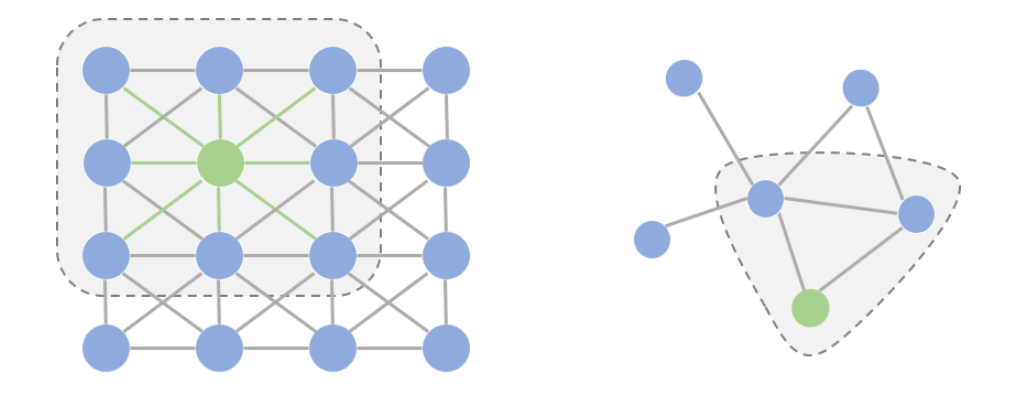GCN## 图卷积起缘2. 欧式空间中的卷积操作实际上是用固定大小可学习的卷积核来抽取像素的特征，比如这里就是抽取绿色结点对应像素及其相邻像素点的特征。但是因为图里的邻居结点不固定，所以传统的卷积核不能直接用于抽取图上结点的特征。

1. 提出一种方式把非欧空间的图转换成欧式空间。

2. 找出一种可处理变长邻居结点的卷积核在图上抽取特征。

## 图卷积框架1. Convolution Layer 1里，对每个结点的邻居都进行一次卷积操作，并用卷积的结果更新该结点；

2. 经过激活函数如ReLU

3. 再过一层卷积层Convolution Layer 2与一层激活函数；

4. 重复1~3步骤，直到层数达到预期深度。

### 卷积操作

#### 空域卷积(Spatial Convolution)#### 频域卷积(Spectral Convolution)

##### 傅里叶变换(Fourier Transform)

FT会将一个在空域(或时域)上定义的函数分解成频域上的若干频率成分。换句话说，傅里叶变换可以将一个函数从空域变到频域。用 $F$ 来表示傅里叶变换的话，这里有一个很重要的恒等式：

$f$ 经过傅里叶变换后 $\hat{f}$ 如下所示：

$\Delta$ 作用在 $e^{-2 \pi i x t}$ 满足上述特征向量的定义：

$\Delta$ 即为 $-4 \pi^2 x^2$，注意这里 $t$ 是变量，$x$ 是常量。本质上，傅里叶变换是将$f(t)$映射到了以$\left\{e^{-2 \pi i x t}\right\}$为基向量的空间中。

##### 图上的傅里叶变换

$I_N$ 为单位矩阵，$D$ 为度矩阵，$A$ 为领阶矩阵，$u$ 是特征向量，$\lambda$ 是特征值。

##### 推导

$\tilde{\Lambda}=\frac{2}{\lambda_{\max }} \Lambda-I_{N}$ ，$\theta^{\prime} \in \mathbb{R}^{K}$ 是Chebyshev系数。切比雪夫多项式是递归定义的：$T_k(x) = 2xT_{k-1}(x) - T_{k-2}(x), T_0(x) = 1, T_1(x) = x$ 。

$\tilde{L}=\frac{2}{\lambda_{max}} L-I_{N}$，因为$\left(U \Lambda U^{\top}\right)^{k}=U \Lambda^{k} U^{\top}$

$\theta=\theta_{0}^{\prime}=-\theta_{1}^{\prime}$，$I_{N}+D^{-\frac{1}{2}} A D^{-\frac{1}{2}}$ 的特征值取值范围现在是 $[0, 2]$ 。为了防止误差在反向传播的过程中出现梯度弥散，将该式进行归一化：

$\Theta \in \mathbb{R}^{C \times F}$ 是可训练的卷积核参数。